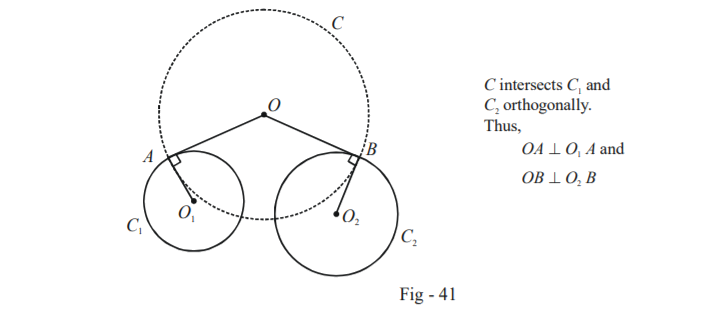# Examples On Common Chords And Radical Axes Set-1

Go back to  'Circles'

Example - 32

A circle $$C$$ passes through $$(1, 1)$$ and cuts the following two circles orthogonally:

\begin{align}&{S_1}:{x^2} + {y^2} - 8x - 2y + 16 = 0\\&{S_2}:{x^2} + {y^2} - 4x - 4y - 1 = 0\end{align}Find the equation of $$C$$.

Solution: We assume the equation of $$C$$ to be

$S:{x^2} + {y^2} + 2gx + 2fy + c = 0$

Applying the condition of orthogonality of $$S$$ with $${S_1}$$ and $${S_2},$$ we obtain:

\begin{align}{\rm\bf{with}}\;{S_1}: \qquad \qquad &2( - 4g - f) = c + 16\\\Rightarrow \qquad &8g + 2f + c = -16 \qquad \dots(1)\\{\rm\bf{with}}\;{S_2}:\qquad \qquad &2( - 2g - 2f) = c - 1\\\Rightarrow \qquad &4g + 4f + c = 1\qquad \quad \; \dots( 2)\end{align}

The third condition can be obtained using the fact that $$C$$ passes through $$(1, 1)$$:

\begin{align}\,\,\,\,\,\,\,\,\,\,\,\,\,\,\,\,\,{1^2} + {1^2} + 2g(1) + 2f(1) + c = 0\\\Rightarrow \qquad 2g + 2f + c = - 2\qquad\qquad\qquad...(3)\end{align}

Solving (1), (2) and (3), we obtain

$g = - \frac{7}{3},\,\,\,f = \frac{{23}}{6},\,\,\,c = - 5$

Thus, the equation of the circle $$C$$ is

$S:3{x^2} + 3{y^2} - 14x + 23y - 15 = 0$

Example - 33

Prove that the locus of the centres of the circles cutting two given circles orthogonally is their radical axis.

Solution: This assertion means that any circle cutting two given circles orthogonally will have its centre lying on the radical axis of the two given circles.

Assume the two fixed given circles to have the following equations:

\begin{align}{S_1}:{x^2} + {y^2} + 2{g_1}x + 2{f_1}y + {c_1} = 0\\{S_2}:{x^2} + {y^2} + 2{g_2}x + 2{f_2}y + {c_2} = 0\end{align}

Let the variable circle be $$S:{x^2} + {y^2} + 2gx + 2fy + c = 0$$ so that its centre is $$( - g, - f)$$ whose locus we wish to determine.

Applying the condition for orthogonality, we obtain

$2(g{g_1} + f{f_1}) = c + {c_1}\,\,\,\,\,\,\,\,\,\,\,\,\,\,\,...(1)$

$2(g{g_2} + f{f_2}) = c + {c_2}\,\,\,\,\,\,\,\,\,\,\,\,\,\,\,\,\,\,\,...( 2)$

By (1) – (2), we obtain

$2g({g_1} - {g_2}) + 2f({f_1} - {f_2}) = {c_1} - {c_2}$

Using $$(x,y)$$ instead of $$( - g, - f),$$ we obtain the locus as $$2x({g_1} - {g_2}) + 2y({f_1} - {f_2}) + ({c_1} - {c_2}) = 0$$ which is the same as the equation of the radical axis, $${S_1} - {S_2} = 0.$$ i.e.

We can also prove this assertion of this question in a very straightforward manner using a pure geometric approach. Let $$C$$ be a circle intersecting the two given circles $${C_1}$$ and $${C_2}$$ orthogonally as show in the figure below:$$OA \bot {O_1}A$$ and $$OB \bot {O_2}B$$ implies that $$OA$$ and $$OB$$ are simply the tangents drawn from $$O$$ to $$\,{C_1}$$ and $${C_2}$$ . Since $$OA$$ and $$OB$$ are the radii of the same circle $$C$$, we have $$OA = OB.$$

Thus, $$O$$ is a point such that tangents drawn from it to the two circles $${C_1}$$ and are equal in length, which implies that $$O$$ lies on the radical axis of $${C_1}$$ and $${C_2}$$.

From this property, a straightforward corollary follows. For three fixed circles, a circle with centre at the radical centre and radius equal to the length of the tangent from it to any of the circles will intersect all the three circles orthogonally. As an exercise, find the equation to the circle $$C$$ cutting the following three circles orthogonally:

$\begin{gathered} {C_1}:{x^2} + {y^2} - 2x + 3y - 7 = 0 \hfill \\ {C_2}:{x^2} + {y^2} + 5x - 5y + 9 = 0 \hfill \\ {C_3}:{x^2} + {y^2} + 7x - 9y + 29 = 0 \hfill \\\end{gathered}$